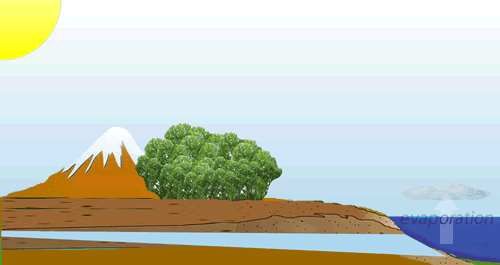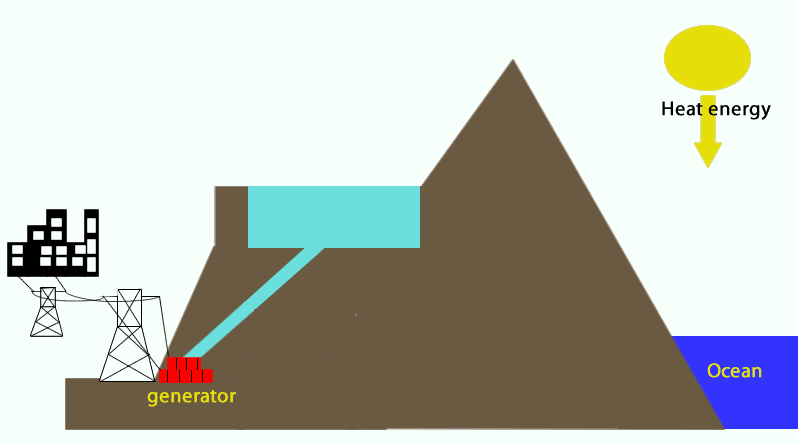Energy conversion of a hydro-electric power station Consider the animation on the right. 1) What is the "Water Cycle"? 2) Where does the energy for evaporation and transpiration come from? 3) What is the difference between transpiration and evaporation? 4) How is the water cycle manipulated to produce electric power?5) Consider the animation below. Describe the energy conversions necessary to produce hydro-electricity starting from water in the oceans.6) From looking at the animation, what is gravitational potential energy? 7) How do the power stations convert gravitational potential energy into kinetic energy? 8) A generator converts electrical energy into gravitational potential energy kinetic energy into electrical energy solar energy into heat energy none of the above 9) Compare the energy conversions between an hydroelectric power plant and a coal fired power plant. What are the similarities and the differences?